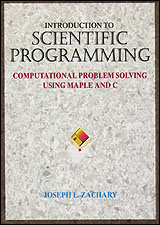### Introduction to Scientific Programming Computational Problem Solving Using: Maple and C Mathematica and C

Author:
Joseph L. Zachary
Online Resources:
Maple/C Version
Mathematica/C Version

# Numerical Integration Tutorial

In this tutorial we will explore the rectangular and trapezoidal methods for numerical integration that are discussed in Chapter 15.

## Simulation

We will be using a graphical simulation of numerical integration methods throughout this tutorial. You can start it by clicking on the following button.

A Java applet should appear here

The simulation window that appears will display a graph with the x- and y-axes in blue and a function in red. In the initial display, the function will be sin(x).

There will also be a pair of vertical white lines at either end of the graph, which represent the limits of integration. In the initial display, the limits will be at -7 and 7.

The problem is to find the area that lies between the red curve and the x-axis and is bounded on either side by the white lines. The simulation can determine the area analytically, and the area is displayed as the "Actual Value" toward the bottom of the simulation window. Notice that this area is zero. This is because area that lies beneath the x-axis is treated as "negative" area and, in the case of sin(x), exactly cancels out the "positive" area above the x-axis.

You can use the mouse to drag the two limit lines back and forth. As you do so, the calculated area will change.

Now use the Method menu to select the rectangular method. Ten green rectangles will be superimposed on the graph. The areas of these rectangles can be calculated and summed to obtain an approximation to the area between the curve and the x-axis. The actual value, the calculated value, and the difference between them will be displayed at the bottom of the simulation window.

You can use the control beneath the graph to change the number of rectangles being used. Notice that as the number of rectangles increases, the amount of error tends to decrease.

Experiment with using the rectangular method to approximate the area beneath the graphs of several different functions. You can use the Function menu to choose new functions.

Also experiment with using either the midpoint or the trapezoidal method to perform the same approximations. You can use the Method menu to choose a new method. How do the three methods compare with each other?